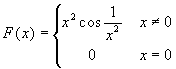www.batmath.it

### Antiderivatives and Riemann integrals: some observations

The fundamental theorem of calculus sets a link between the concept of antiderivative and of Riemann integral:

• the Riemann integralis an antiderivative of the continuous function f;
• given an antiderivative G(x) of a continuous function f we have:.

Despite this important link, the two concepts remain quite different: the algorithm used to calculate antiderivatives produces a class of functions; the one used to calculate Riemann integrals produces a real number.

Besides this we want to point out the following facts.

• The set of all integral functions of a given continuous function f is, in general, a subset of the set of all antiderivatives. An integral function is always zero at the starting point:, while an antiderivative may be always strictly positive. For example the function F(x)=x2+1 is an antiderivative of f(x)=2x, but it is nowhere zero: this means that it can't be an integral function.
• A function that has a jump discontinuity can't have antiderivatives, while it may be integrable. The simplest examples are  step functions.
• The integral functions of a non continuous function usually are not antiderivatives. For example, but the function |x| is not an antiderivative, as it is not differentiable in zero. Observe that this equality is an alternative definition of the absolute value function.
• An unbounded function can't be Riemann integrable, while it may have antiderivatives. For an example try to find the derivative, f(x), of. As f is unbounded it is certainly not Riemann integrable, but obviously it has an antiderivative, the function F itself!
first published on january 07 2003 - last updated on september 01 2003